# Simulating Designs Using SPICE

### General Approach

In order to verify the theoretical results, debug the related design software and evaluate potential designs, a collection of SPICE simulations of various systems will be performed in this section and the results presented. These simulations will all use the Dayton RSS390HF-4 driver, which is well suited to large vented-box subwoofers having a -3dB frequency in the neighborhood of 20 Hz. Simulations of frequency response and displacement will be performed. First, an unassisted system will be examined without any external filter, then with four different add-on high-pass filters. A collection of assisted alignments of order n from 5 through 8 will be designed, analyzed and compared to the unassisted alignments with add-on high-pass filters to determine if there is any advantage to incorporating the filters into the design process itself. All the possible alignment classes are evaluated for each n value of the assisted alignments: one fifth-order, three sixth-order, three seventh-order and six eighth-order. In some cases, alignments with little or no practical usage will arise. This will be noted when found.

### Schematic and Description of Typical Simulation

The simulation technique is a variant of the approach used by Leach , except that it uses the free LTspice simulator from Linear Technology. Leach's approach includes the effects of more complex phenomena than those usually considered, including mutual coupling between the port and driver, as well as a more complex model of radiation impedance than the acoustic mass loading that's typically used. In contrast, the simulations provided here are structured so that the transfer function of the loudspeaker plus box is identical to G(s) given in Small  and here in (1), with transfer function coefficients given by (14) - (17). The schematic of a typical simulation is shown below in Figure 3.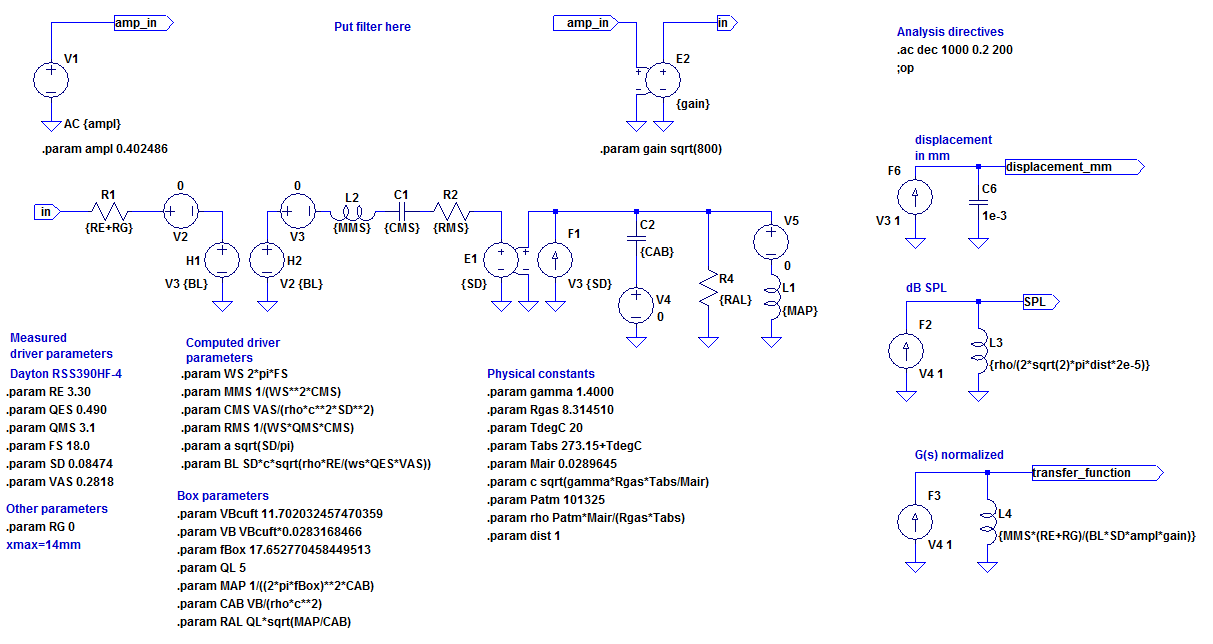Figure 3. Simulation Schematic of a Typical Unassisted Vented Box Alignment

The circuit keeps the electrical, mechanical and acoustical portions separate to allow for easy probing of different types of physical quantities without resorting to transformations between the electrical, mechanical and acoustical domains. Voltage source `V1` represents the preamp output voltage, while voltage-controlled voltage source `E2` represents a power amplifier with THX-compliant gain. For assisted alignments, a filter (not shown) is placed between `V1` and `E2`. When such a filter is used, its transfer function is entered as a `Laplace` expression for the scale factor of a voltage-controlled voltage source. The Thiele-Small parameters of the driver are entered as `.param` statements under "Measured driver parameters". The box volume in cubic feet, box tuning frequency in Hz and box Q are entered as `VBcuft`, `fBox` and `QL` respectively. The parameter `dist` is the distance in meters, used to compute the dB SPL. All parameters are in MKS units unless they explicitly have a units suffix as in e.g. `VBcuft`.

To ensure that G(s) has the form of a high-pass filter function, the voice coil inductance is neglected. The effective mechanical mass MMS is really the sum of the mechanical mass MMD of the driver diaphragm assembly itself, plus the acoustic mass loads on the front and back of the driver reflected through the transformer to the mechanical section. This simplifies the equivalent circuit and the computations of the element values from the driver Thiele-Small parameters. It can be seen in Figure 3 that reflecting all electrical and mechanical elements to the acoustical side of the circuit will result in an acoustical analogous circuit of identical form to Figure 1. Given a correct computation of the element values, a direct, one-to-one relationship is established between the synthesis and analysis, allowing for verification of both the design equations and the software code for solving them.

On the right side of Figure 3, three test voltages are provided, denoted by `displacement_mm`, `SPL` and `transfer_function`. These represent the peak displacement of the cone in millimeters, the SPL at distance `dist`, and the transfer function G(s) (or H(s) if it is an assisted alignment) respectively. The voltage representing the transfer function is scaled so as to be constant with variations of the source amplitude `ampl` and amplifier gain value `gain`.

The LTspice simulations for all examples can be downloaded here. The Matlab/Octave scripts used for finding the design parameters can be downloaded here. No Matlab/Octave scripts are present for examples 2-5 as these use only the SPICE simulator to determine the filter parameters.

## Unassisted Alignments With Add-On High-Pass Filters

### Example 1: Frequency Response and Cone Displacement vs. Frequency of Unassisted Alignment

When a constant-amplitude frequency sweep is applied in the simulation of Figure 3, the transfer function magnitude and displacement in mm vs. frequency are as shown in Figure 4 below. The frequency span is 0.2Hz to 200Hz. The minimum frequency of the sweep is quite low in order to demonstrate the increase in displacement at very low frequencies. All remaining simulations will have some kind of high-pass filter in the system, making the system behavior near DC irrelevant. These remaining simulations will have plots whose frequency span is 2Hz to 200Hz.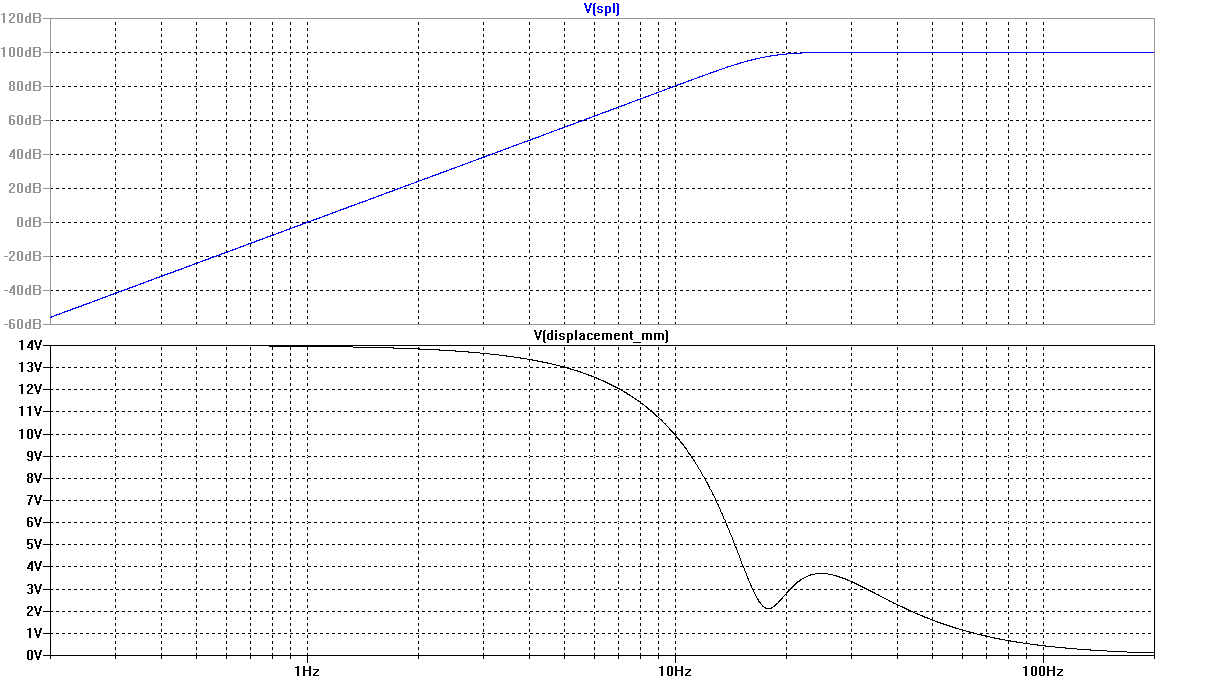Figure 4. Frequency Response and Cone Displacement vs. Frequency of Unassisted Alignment

This system was designed using the Matlab/Octave design software for unassisted alignments. It is a Chebyshev alignment with a box tuning frequency of 17.65Hz, just below the 18Hz free-air resonance of the driver. Box volume is 11.7ft3, ripple is negligible and the -3dB frequency is 17.6Hz.

The amplitude shown on the left axis of the `displacement_mm` plot indicates units of Volts, but as explained earlier, it is scaled to indicate peak cone displacement in mm. The source amplitude has been adjusted so the maximum peak displacement, which occurs at very low frequencies, is 14 mm, the maximum allowable displacement xmax of the driver.

#### The Benefit of a High-Pass Filter

Figure 4 shows a number of interesting features regarding the displacement and its relationship to the frequency response. Near the box tuning frequency, the displacement reaches a local minimum. At a frequency slightly above the box tuning frequency, a local maximum of displacement is seen, but the amplitude response is still reasonably flat at that frequency. Below the box tuning frequency, the amplitude drops off at 24dB/oct, yet the displacement markedly increases as frequency decreases. Clearly, a properly chosen high-pass filter would allow the preamp output voltage to sweep at a much higher amplitude before the worst-case displacement of the cone reaches xmax.

We might expect the displacement behavior with such a high-pass filter to be as follows. At very low frequencies, the displacement would approach zero, then as frequency increases, the displacement would increase, finally reaching a peak at a frequency somewhat below the box tuning frequency. As frequency increases further, the displacement would decrease again, reaching a minimum near the box tuning frequency, then rise again to reach another peak at a frequency close to the existing one near 25Hz. If the lower-frequency peak were lower in amplitude than the higher-frequency one, this would indicate overzealous filtering which would unnecessarily increase the lower -3dB frequency of the system. If the lower-frequency peak were higher in amplitude than the higher-frequency one, this might provide less protection from excessive displacement than we'd like. For a given filter, the criterion used to adjust the filter cutoff frequency will be to achieve equal-amplitude displacement peaks. Then the preamp output amplitude will be adjusted so the maximum displacement over frequency is xmax.

Two figures of merit will be used. The first will be the system -3dB frequency when the filter, if any, is present. The second is the reference SPL. The reference SPL is defined as the SPL at 1m distance with the preamp output amplitude chosen for a maximum displacement of xmax over frequency as described above. The reference frequency at which this SPL is simulated will be 200Hz, where the response has become flat. If instead one were to pick the reference frequency as the one for which maximum displacement is reached, a system without a high-pass filter would have a reference SPL of negative infinity at DC. Since this is is not a useful result, the SPL in the region of flat response will be used. This reference SPL is not the actual maximum SPL achievable at 200Hz because the system is power-limited, not displacement-limited there. Choosing the reference SPL in this way might be subject to some debate. However, one system design strategy is to pick the amplifier maximum power so that the amplifier just clips at a voltage level corresponding to the worst-case maximum displacement of the subwoofer cone. For this design approach, the definition of reference SPL chosen here is a reasonable representation of achievable real-world performance.

#### Performance Summary for Example 1

The unassisted alignment with no high-pass filter has the following data:
VB = 11.7ft3
fB = 17.7Hz
System f3 = 17.4Hz
Reference SPL = 100.2dB
dB ripple < 0.01dB
k value = 0.9473

### Example 2: Unassisted Alignment with Second-Order Butterworth High-Pass Filter

A second-order Butterworth high-pass filter was added to the system of Example 1 and the filter's -3dB frequency adjusted for equal-amplitude displacement peaks. A plot of the transfer function and displacement is shown below in Figure 5.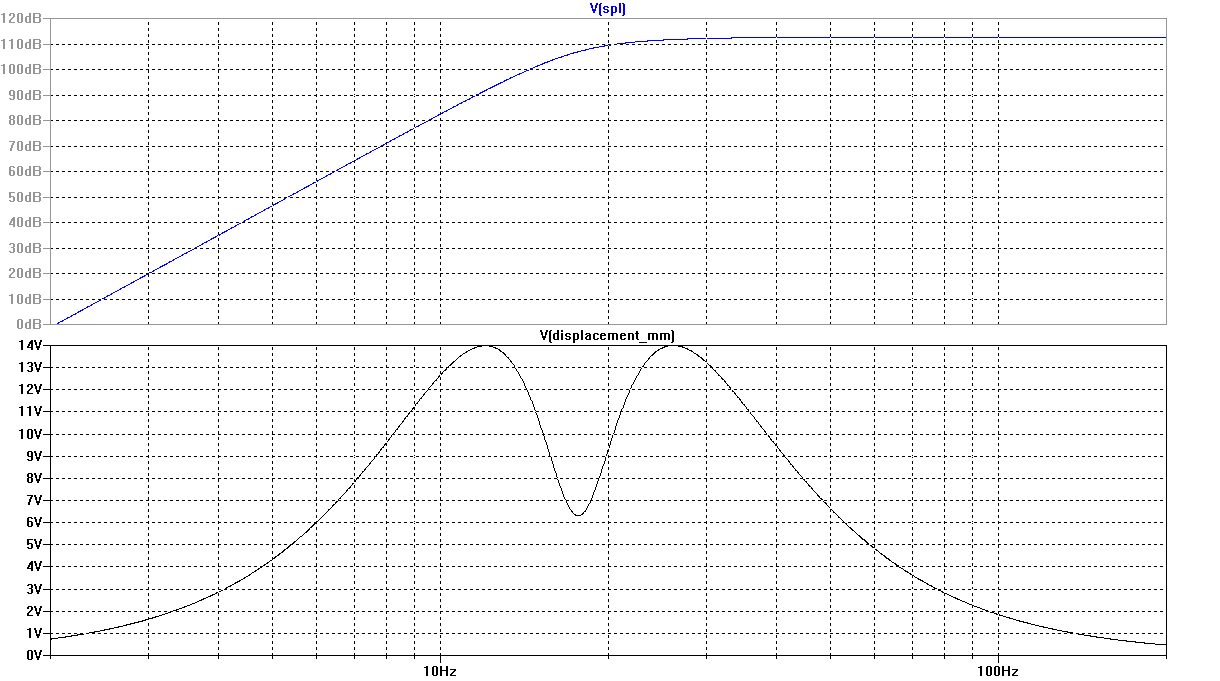Figure 5. Frequency Response and Cone Displacement vs. Frequency of Unassisted Alignment with Second-Order Butterworth High-Pass Filter

#### Performance Summary for Example 2

The unassisted alignment with second-order Butterworth high-pass filter has the following data:
VB = 11.7ft3
fB = 17.7Hz
Filter f3 = 17.7Hz
System f3 = 20.3Hz
Reference SPL = 112.7dB
dB ripple < 0.01dB
k value = 0.9473

The data show an undesirable increase of the system -3dB frequency from 17.4Hz to 20.3Hz combined with a very desirable and substantial 12.5dB increase in the reference SPL. The DC value of the displacement function of the unassisted system without filter is more than 4 times the displacement at the local maximum near 25Hz. Setting the DC displacement to zero with the high-pass filter allows the preamp output amplitude to increase such that the secondary displacement peak near 25Hz reaches the driver xmax value of 14mm. One might expect an increase in the order of the high-pass filter to have negligible effect on the reference SPL while decreasing the system -3dB frequency somewhat. The following examples will provide more specific data.

### Example 3: Unassisted Alignment with Third-Order Butterworth High-Pass Filter

A third-order Butterworth high-pass filter was added to the system of Example 1 and adjusted for equal-amplitude displacement peaks. A plot of the transfer function and displacement is shown below in Figure 6.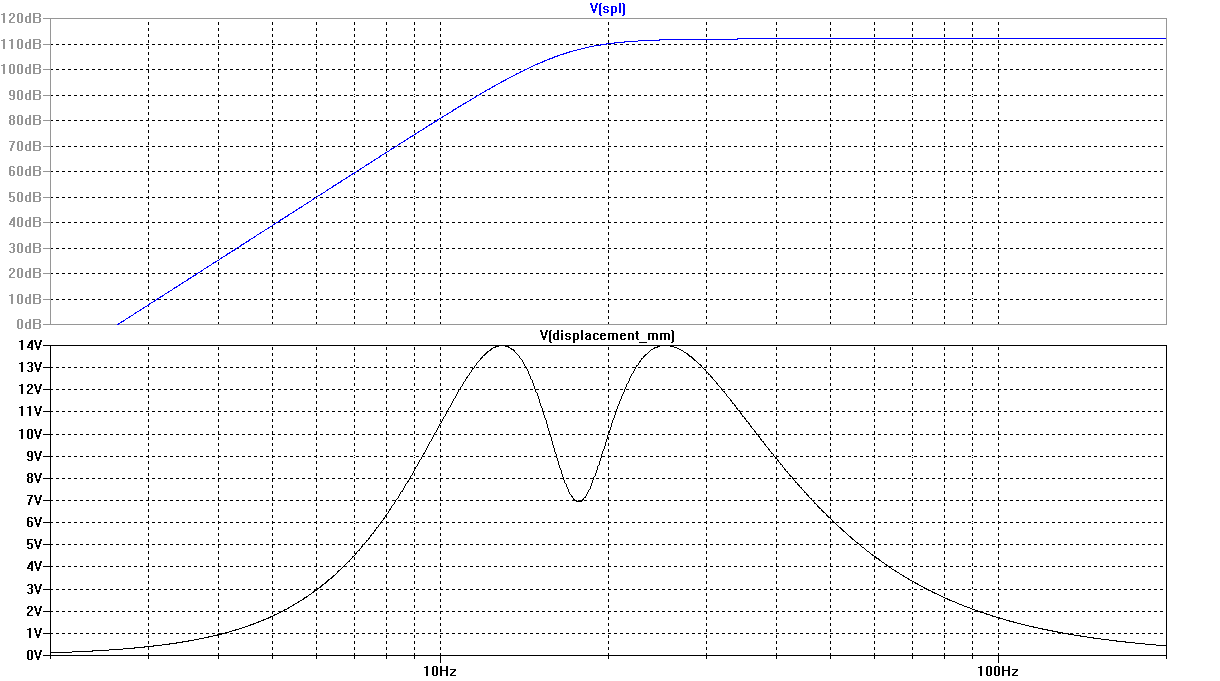Figure 6. Frequency Response and Cone Displacement vs. Frequency of Unassisted Alignment with Third-Order Butterworth High-Pass Filter

#### Performance Summary for Example 3

The unassisted alignment with third-order Butterworth high-pass filter has the following data:
VB = 11.7ft3
fB = 17.7Hz
Filter f3 = 15.2Hz
System f3 = 18.6Hz
Reference SPL = 112.0dB
dB ripple < 0.01dB
k value = 0.9473

### Example 4: Unassisted Alignment with Fourth-Order Butterworth High-Pass Filter

A fourth-order Butterworth high-pass filter was added to the system of Example 1 and adjusted for equal-amplitude displacement peaks. A plot of the transfer function and displacement is shown below in Figure 7.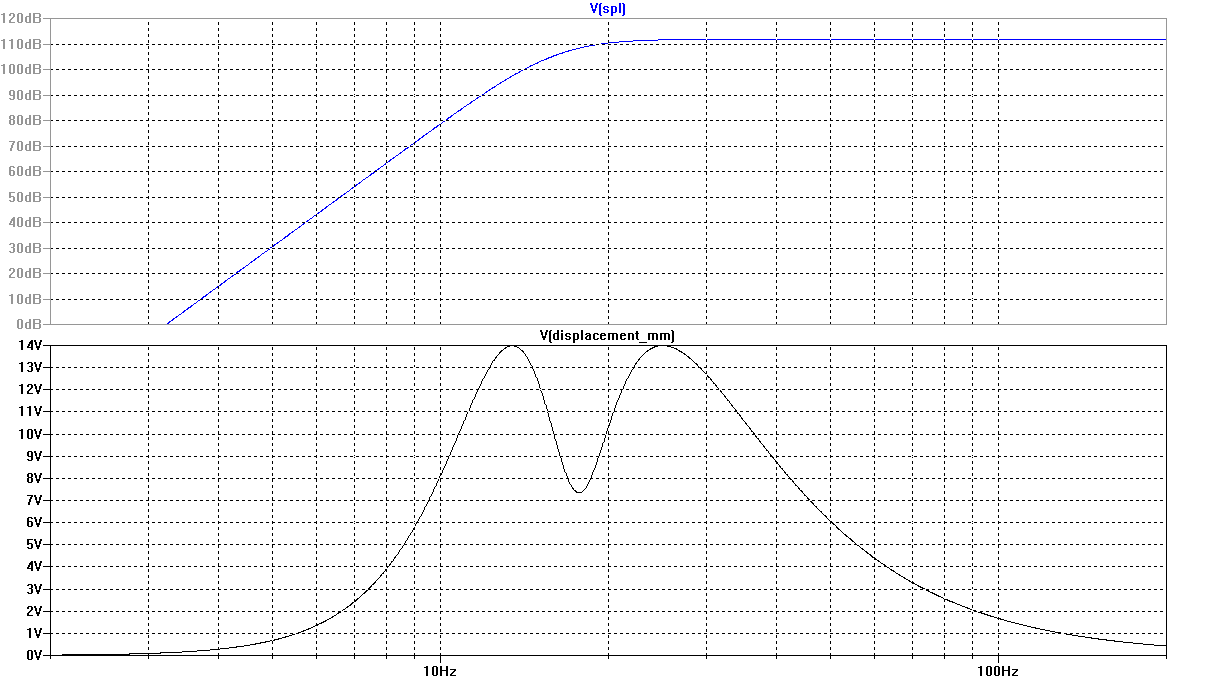Figure 7. Frequency Response and Cone Displacement vs. Frequency of Unassisted Alignment with Fourth-Order Butterworth High-Pass Filter

#### Performance Summary for Example 4

The unassisted alignment with fourth-order Butterworth high-pass filter has the following data:
VB = 11.7ft3
fB = 17.7Hz
Filter f3 = 14.6Hz
System f3 = 18.1Hz
Reference SPL = 111.9dB
dB ripple < 0.01dB
k value = 0.9473

### Example 5: Unassisted Alignment with Third-Order Inverse Chebyshev High-Pass Filter

A third-order inverse Chebyshev high-pass filter was added to the system of Example 1 and adjusted for equal-amplitude displacement peaks. The inverse Chebyshev filter is a little-used filter type having a maximally flat response in the passband and equal ripple in the stopband. Depending on flter order, the stopband has at least one notch, the presence of which causes the response in the transition band to drop more rapidly than a typical all-pole filter such as a Butterworth of the same order. Additional information about inverse Chebyshev filters and how to design them for this application can be found in the appendix. A plot of the transfer function and displacement using the inverse Chebyshev filter is shown below in Figure 8.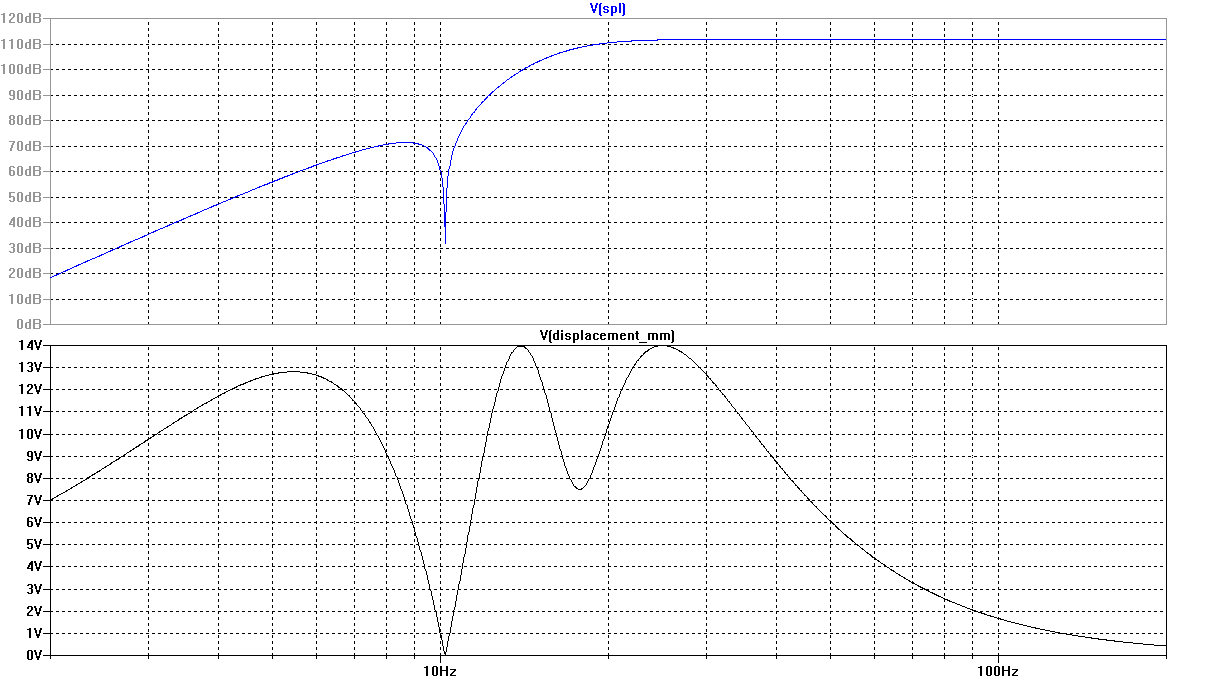Figure 8. Frequency Response and Cone Displacement vs. Frequency of Unassisted Alignment with Third-Order Inverse Chebyshev High-Pass Filter

#### Performance Summary for Example 5

The unassisted alignment with third-order inverse Chebyshev high-pass filter has the following data:
VB = 11.7ft3
fB = 17.7Hz
Filter f3 = 14.4Hz
System f3 = 18.0Hz
Reference SPL = 111.8dB
dB ripple < 0.01dB
k value = 0.9473

It can be seen that the third-order inverse Chebyshev filter outperforms the fourth-order Butterworth by a very slight amount. However, the additional filter complexity caused by the need for a pair of j-axis zeros in the transfer function may make this solution less appealing than filters having all their zeros at the origin.